# Creating high crime sub-tours

I was nerdsniped a bit by this paper, Targeting Knife-Enabled Homicides For Preventive Policing: A Stratified Resource Allocation Model by Vincent Hariman and Larry Sherman (HS from here on).

It in, HS attempt to define a touring schedule based on knife crime risk at the lower super output area in London. So here are the identified high risk areas: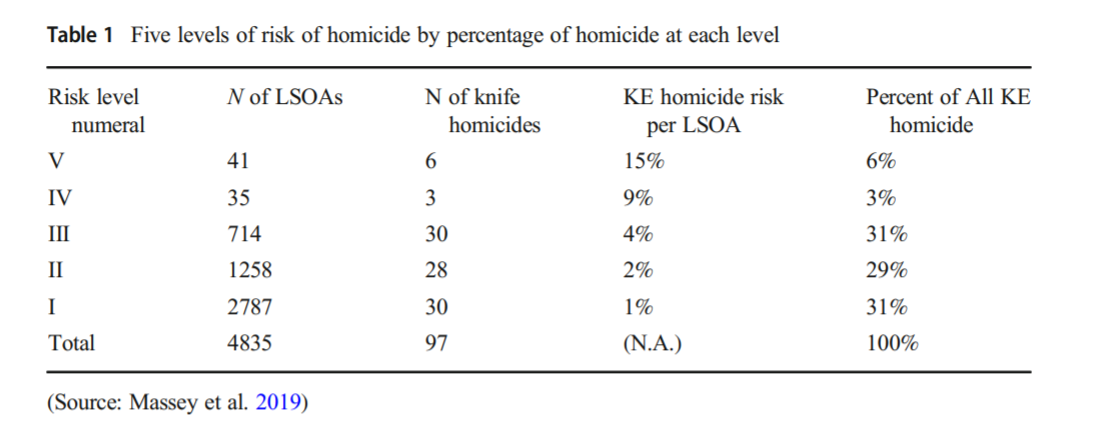And here are HS’s suggested hot spot tours schedule.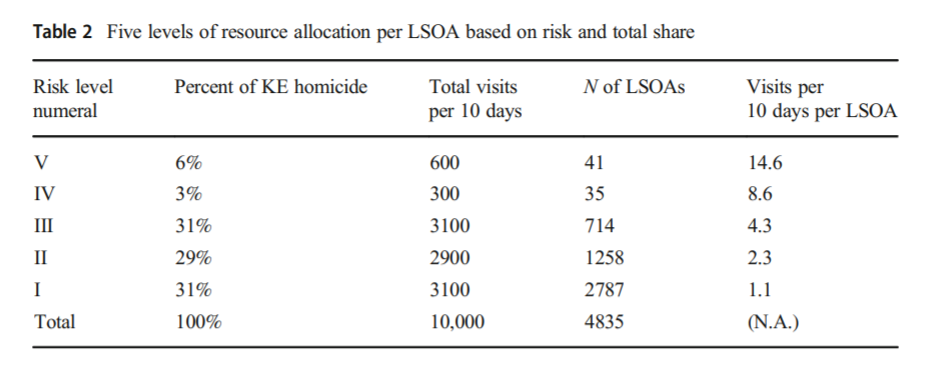This is ad-hoc, but an admirable attempt to figure out a reasonable schedule. As you can see in their tables, the ‘high’ knife crime risk areas still only have a handful of homicides, so if reducing homicides is the objective, this program is a bit dead in the water (I’ve written about the lack of predictive ability of the model here).

I don’t think defining tours to visit everywhere makes sense, but I do think a somewhat smaller in scope question, how to figure out geographically informed tours for hot spot areas does. So instead of the single grid cell target ala PredPol, pick out multiple areas to visit for hot spots. (I don’t imagine the 41 LSOA areas are geographically contiguous either, e.g. it would make more sense to pick a tour for areas connected than for areas very far apart.)

Officers don’t tend to like single tiny areas either really, and I think it makes more sense to widen the scope a bit. So here is my attempt to figure those reasonable tours out.

# Defining the Problem

The way I think about that problem is like this, look at the hypothetical diagram below. We have two choices for the hot spot location we are targeting, where the crime counts for locations are noted in the text label.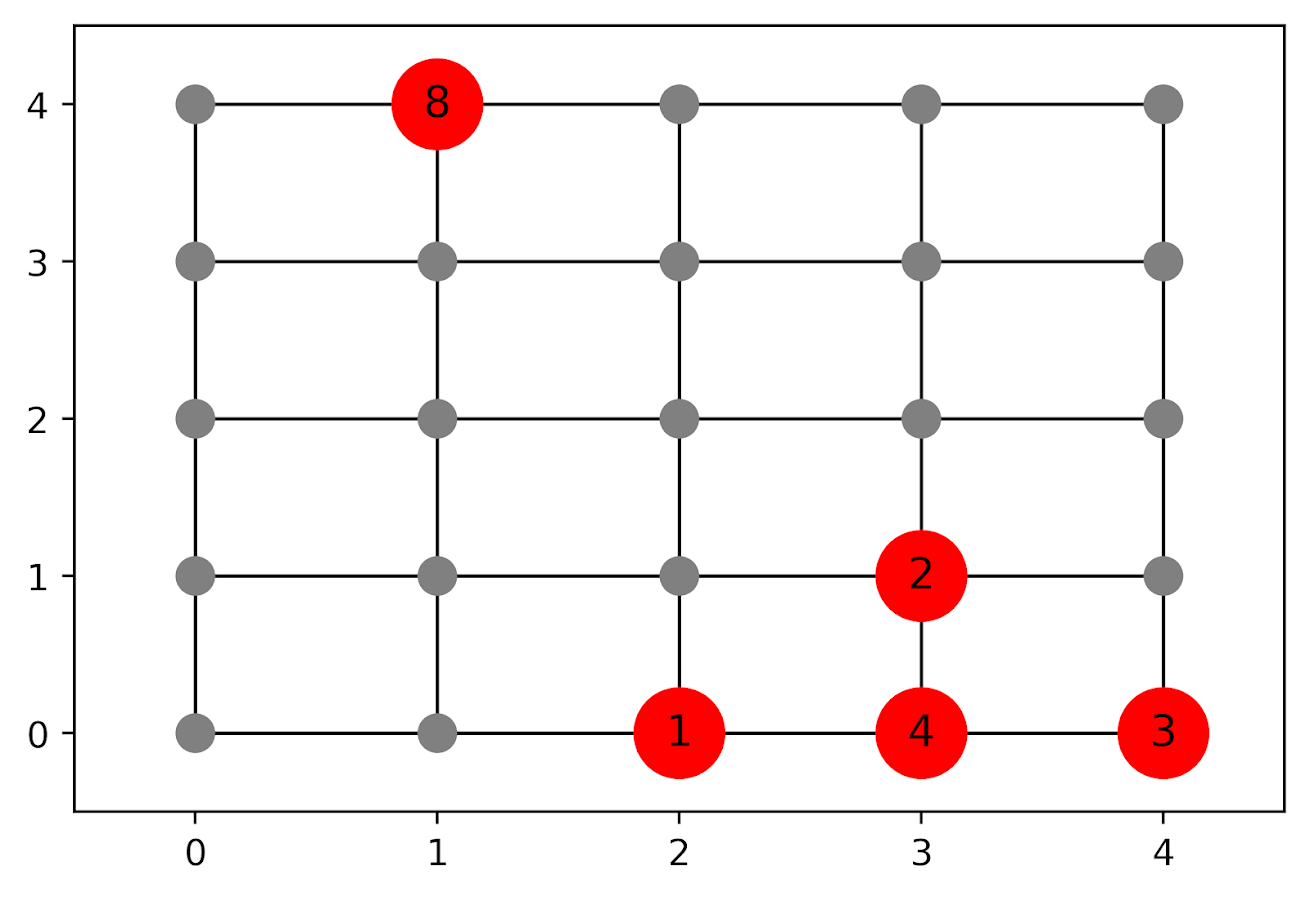In the select the top hot spot (e.g. PredPol) approach, you would select the singlet grid cell in the top left, it is the highest intensity. We have another choice though, the more spread out hot spot in the lower right. Even though it is a lower density, it ends up capturing more crime overall.

I subsequently formulated an integer linear program to try to tackle the problem of finding good sub-tours through the graph that cumulatively capture more crime. So with the above graph, if I select two subtours, I get the results as (where nodes are identified by their `(x,y)` position):

• `['Begin', (1, 4), 'End']`
• `['Begin', (4, 0), (4, 1), (3, 1), (3, 0), (2, 0), 'End']`

So it can select singlet areas if they are islands (the `(1,4)` area in the top left), but will grow to wind through areas. Also note that the way I have programmed this network, it doesn’t skip the zero area `(4,1)` (it needs to go through at least one in the bottom right unless it doubles back on itself).

I will explain the meaning of the begin and end nodes below in my description of the linear program. It ends up being sort of a mash-up of traveling salesman type vehicle routing and min cost max flow type problems.

# The Linear Program

The way I think about this problem formulation is like this: we have a directed graph, in which you can say, OK I start from location A, then can go to B, than go to C. In my set of decision variables, I have choices that look like this, where the first subscript denotes the from node, and the second subscript denotes the to node.

``````D_ab := node a -> node b
D_bc := node b -> node c``````

etc. In our subsequent linear program, the destination node is the node that we calculate our cumulative crime density statistics. So if node B had 10 crimes and 0.1 square kilometers, we would have a density of 100 crimes per square kilometer.

Now to make this formulation work, we need to add in a set of special nodes into our usual location network. These nodes I call ‘Begin’ and ‘End’ nodes (you may also call them source/sink nodes though). The begin nodes all look like this:

``````D_{begin},a
D_{begin},b
D_{begin},c``````

So you do that for every node in your network. Then you have End nodes as well, e.g.

``````D_a,{end}
D_b,{end}
D_c,{end}``````

In this formulation, since we are only concerned about the crime stats for the to node, not the from node, the Begin nodes just inherit the crime density stats from the original node data. For the end nodes though, you just set their objective value stats to zero (they are only relevant to define the constraints).

Now here is my linear program formulation:

``````Maximize
Sum [ D_ij ( CrimeDensity_j - DensityPenalty_j ) ]

Subject To:

1. Sum( D_in for each neighbor of n ) <= 1,
for each original node n
2. Sum( D_in for each neighbor of n ) =  Sum( D_ni for each neighbor of n ),
for each original node n
3. Sum( D_bi for each begin node ) = k routes
4. Sum( D_ie for each end node ) = k routes
5. Sum( D_ij + D_ji ) <= 1, for each unique i,j pair
6. D_ij is an element of {0,1}``````

Constraint 1 is a flow constraint. If a node has an incoming edge set to one, it cannot have any other incoming edge set to one (so a location can only be chosen once).

Constraint 2 is a constraint that says if an incoming node is selected, one of the outgoing edges needs to be selected.

Constraints 3 & 4 determine the number of k tours/routes to choose in the end. Since the begin/end nodes are special we have k routes going out of the begin nodes, and k routes going into the end nodes.

With just these constraints, you can still get micro-cycles I found. So something like, X -> Z -> X. Constraint 5 (for only the undirected edges) prevents this from happening.

Constraint 6 is just setting the decision variables to binary 0/1. So it is a mixed integer linear program.

The final thing to note is the objective function, I have `CrimeDensity_j - DensityPenalty_j`, so what exactly is DensityPenalty? This is a value that penalizes visiting areas that are below this threshold. Basically the way that this works is that, the density penalty sets an approximate threshold for the minimum density a tour should contain.

I suggest a default of a predictive accuracy index of 10. Where do I get 10 you ask? Weisburd’s law of crime concentration suggests 5% of the areas should contain 50% of the crime, which is a PAI of 0.5/0.05 = 10. In my example with DC data then I just calculate the actual density of crime per unit area that corresponds to a PAI of 10.

You can adjust this though, if you prefer smaller tours of higher crime density you would up the value. If you prefer longer tours decrease it.

This is the best way I could figure out how to trade off the idea of spreading out the targeted hot spot vs selecting the best areas. If you spread out you will ultimately have a lower density. This turns it into a soft objective penalty to try to keep the selected tours at a particular density threshold (and will scoop up better tours if they are available). For awhile I tried to figure out if I could maximize the PAI metric, but it is the case with larger areas the PAI will always go down, so you need to define the objective some other way.

This formulation I only consider linked nodes (unlike the usual traveling salesman in which it is a completely linked distance graph). That makes it much more manageable. In this formulation, if you have `N` as the number of nodes/areas, and `E` is the number of directed edges between those areas, we will then have:

• `2*N + E` decision variables
• `2 + 2*N + E/2` constraints

Generally if you are doing directly connected areas in geographic networks (i.e. contiguity connections), you will have less than 8 (typically more like an average of 6) neighbors per each area. So in the case of the 4k London lower super output areas, if I chose tours I would guess it would end up being fewer than `2*4,000 + 8*4,000 = 40,000` decision variables, and then fewer than that constraints.

Since that is puny (and I would suggest doing this at a smaller geographic resolution) I tested it out on a harder network. I used the data from my dissertation, a network of 21,506 street units (both street segments and intersections) in Washington, D.C. The contiguity I use for these micro units is based on the Voronoi tessellation, so tends to have more neighbors than you would with a strictly road based network connectivity. Still in the end it ends up being a shade fewer than 200k decision variables and 110k constraints. So is a better test for in the wild whether the problem can be feasibly solved I think.

# Example with DC Data

Here I have posted the python code and data used for this analysis, I end up having a nice function that you just submit your network with the appropriate attributes and out pops the different tours.

So I end up doing examples of 4 and 8 subtours based on 2011 violent UCR crime data (agg assaults, robberies, and homicides, no rapes in the public data). I use for the penalty that PAI = 10 threshold, so it should limit tours to approximately that value. It only ends up taking 2 minutes for the model to converge for the 4 tours and less than 2.5 minutes for the 8 tours on my desktop. So it should be not a big problem to up the decision variables to more sub-areas and still be solvable in real life applications.

The area estimates are in square meters, hence the high numbers. But on the right you can see that each sub-tour has a PAI above 10.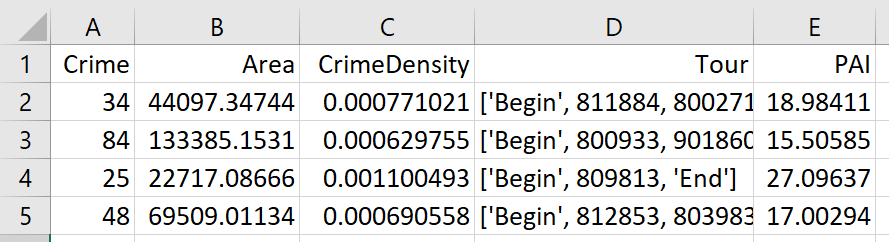Here is an interactive map for you to zoom into each 4 subtour example. Below is a screenshot of one of the subtours. You can see that since I have defined my connected areas in terms of Voronoi tessalations, they don’t exactly follow the street network.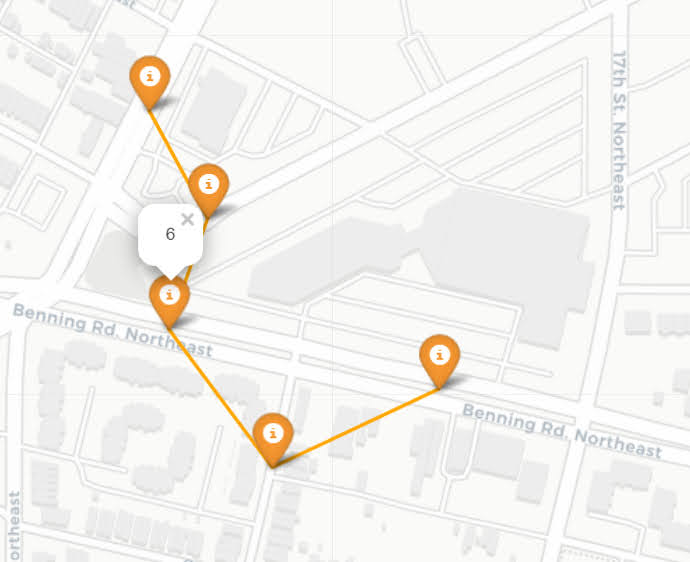For the 8 tour example, it ends up returning several zero tours, so it is not possible in this data to generate 8 sub-tours that meet that PAI >= 10 threshold.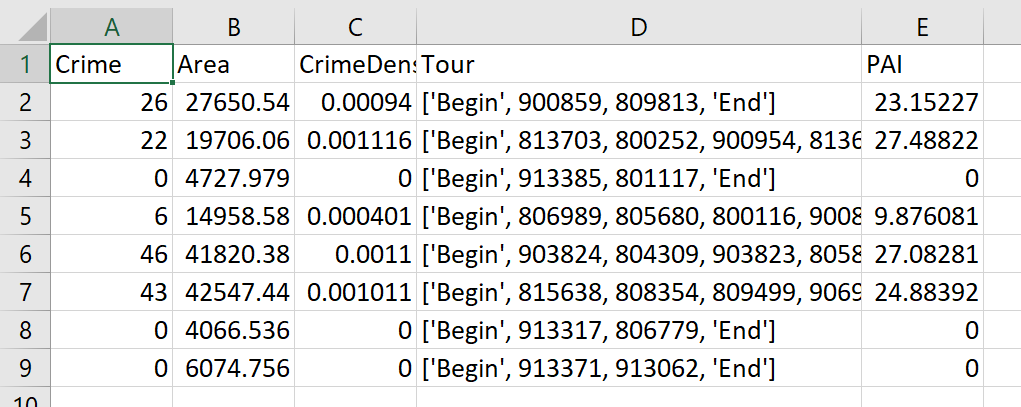You can see that it ends up being the tours have higher PAI values, but lower overall crime counts.

You may think, why does it not pick at least singlet areas with at least one crime? It ends up being that I weight areas here by their area (this formulation would be better with grid cells of equal area, so my objective function is technically `Sum [ D_ij * w_j *( CrimeDensity_j - DensityPenalty_j ) ]`, where w_j is the percent of the total area (so the denominator in the PAI calculation). So it ends up picking areas that are the tiniest areas, as they result in the smallest penalty to the objective function (w_j is tiny). I think this is OK though in the end – I rather know that some of the tours are worthless.

You can also see I get one subtour that is just under the PAI 10 threshold. Again possible here, but should be only slightly below in the worst case scenario. The way the objective function works is that it is pretty tricky to pick out subtours below that PAI value but still have a positive contribution to the overall objective function.

# Future Directions

The main thing I wish I could do with the current algorithm (but can’t the way the linear program is set up), is to have minimum and maximum tour area/length constraints. I think I can maybe do this by adapting this code (I’m not sure how to do the penalties/objectives though). So if others have ideas let me know!

I admit that this may be overkill, and maybe just doing more typical crime clustering algorithms may be sufficient. E.g. doing DBSCAN hot spots like I did here.

But this is my best attempt shake at the problem for now!

# Using Steiner trees to select a subgraph of interest

This is just a quick blog post. A crime analyst friend the other day posed a network problem to me. They had a social network in which they had particular individuals of interest, and wanted to show just a subset of that graph that connected those key individuals. The motivation was for plotting – if you show the entire hairball it can become really difficult to uncover any relationships.

Here is an example gang network from this paper. I randomly chose 10 nodes to highlight (larger red circles), and you can see it is quite hairy. You often want to label the nodes for these types of graphs, but that becomes impossible with so many intertwined nodes.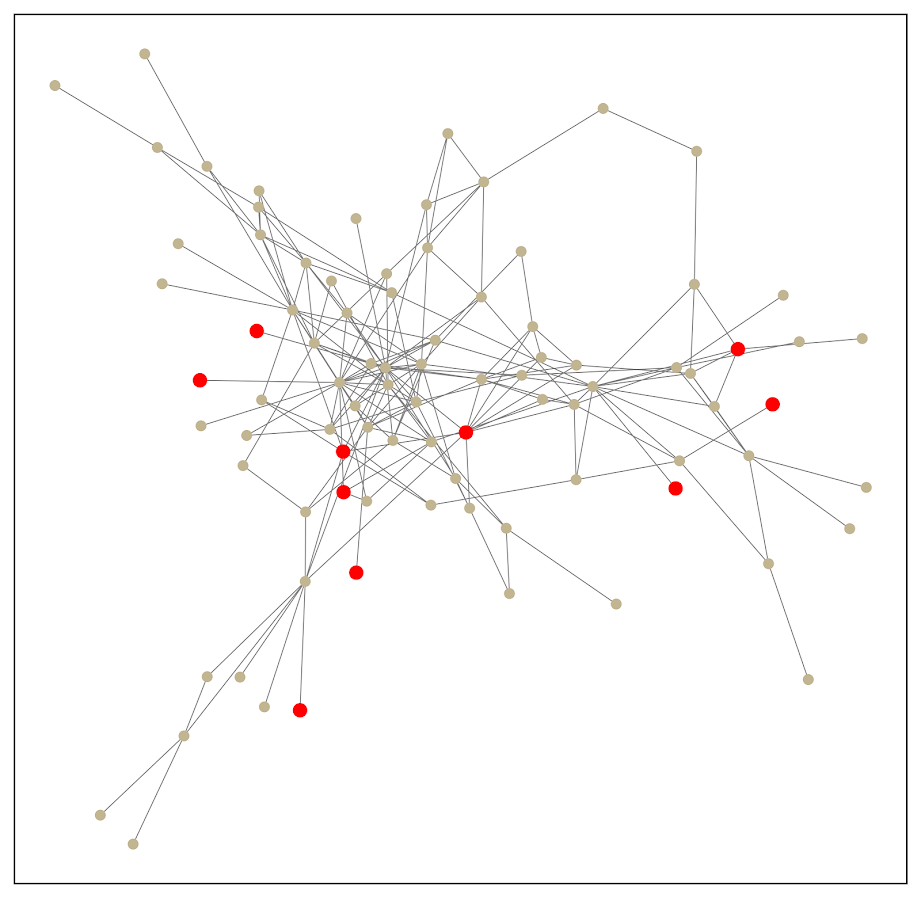One solution to select out a subgraph of the connected bits is to use a Steiner tree. Here is that graph after running the approximate Steiner tree algorithm in networkx (in python).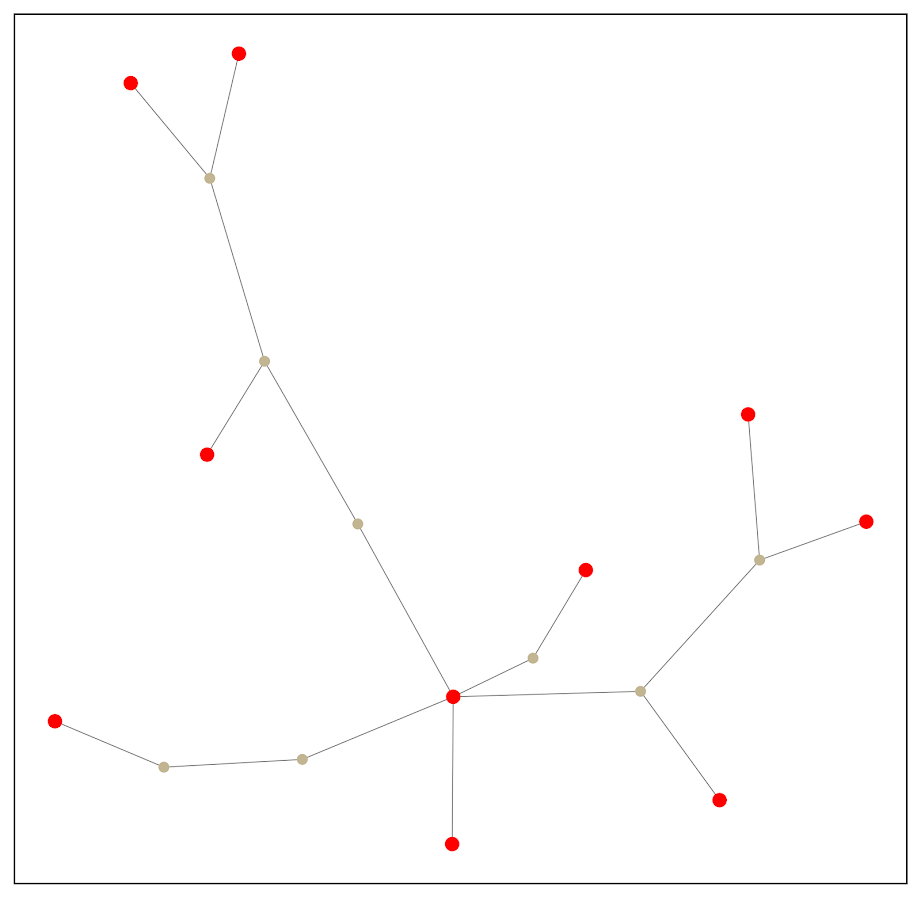Much simpler! And much more space to put additional labels.

I’ve posted the code and data to replicate here. Initially I debated on solving this via setting up the problem as a min-cost-flow, where one of the highlighted nodes had the supply, and the other highlighted nodes had the demand. But this approximate algorithm in my few tests looks really good in selecting tiny subsets, so not much need.

A few things to note about this. It is likely for many dense networks there will be many alternative subsets that are the same size, but different nodes (e.g. you can swap out a node and have the same looking network). A better approach to see connections between interesting nodes may be a betweenness centrality metric, where you only consider the flows between the highlighted nodes.

A partial solution to that problem is to add nodes/edges back in after the Steiner tree subset. Here is an example where I add back in all first degree nodes to the red nodes of interest: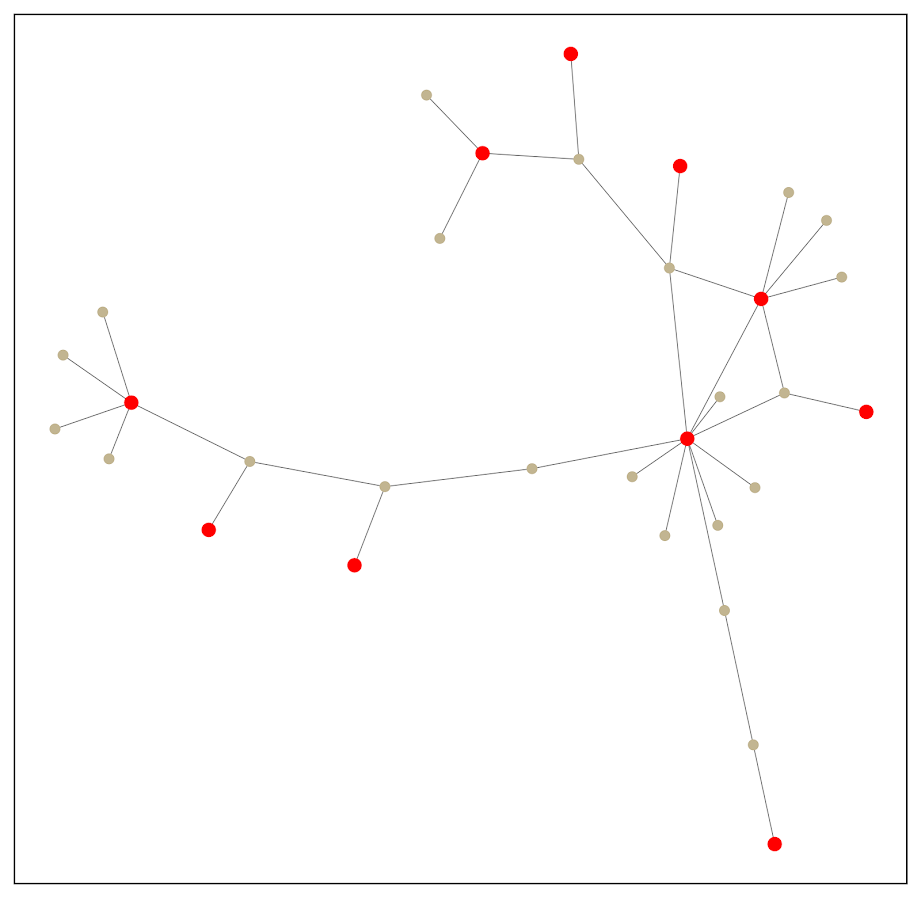So it is still a tiny enough network to plot. This just provides a way to identify higher order nodes of interest that aren’t directly connected to those red nodes.

# Finding the dominant set in a network (python)

My paper, Choosing representatives to deliver the message in a group violence intervention, is now published online at the Justice Evaluation Journal. For those who don’t have access to that journal, here is a link good for 50 e-prints (for a limited time), and here is a pre-print version, and you can always send me an email for the published copy.

I’ve posted Python code to replicate the analysis, including the original network nodes and edges group data. I figured I would go through a quick example of applying the code for others to use the algorithm.

The main idea is that for a focused deterrence initiative, for the call-ins you want to identify folks to spread the deterrence message around the network. When working with several PDs I figured looking at who was called in would be interesting. Literally the first network graph I drew was below on the left — folks who were called in are the big red squares. This was one of the main problem gangs, and the PD had done several call-ins for over a year at this point. Those are not quite the worst set of four folks to call-in based on the topology of the network, but damn close.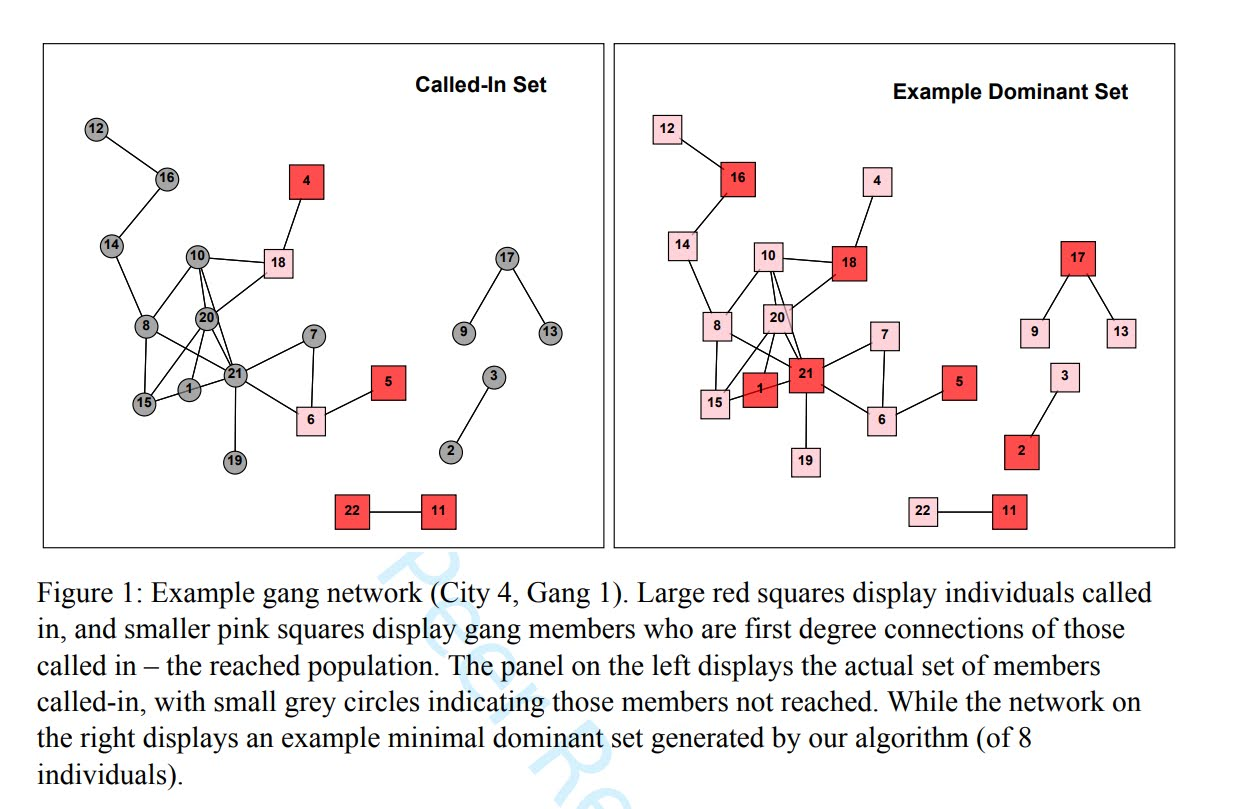But to criticize the PD I need to come up with a better solution — which is the graph on the right hand side. The larger red squares are my suggested call-ins, and they reach everyone within one step. That means everyone is at most just one link away from someone who attended the call-in. This is called a dominant set of a graph when all of the graph is colored in.

Below I give a quicker example using my code for others to generate the dominant set (instead of going through all of the replication analysis). If you are a PD interested in applying this for your focused deterrence initiative let me know!

So first to set up your python code, I import all of the needed libraries (only non-standard is networkx). Then I import my set of functions, named `MyFunctions.py`, and then change the working directory.

``````############################################################
#The libraries I need

import itertools
import networkx as nx
import csv
import sys
import os

#Now importing my own functions I made
locDir = r'C:\Users\axw161530\Dropbox\Documents\BLOG\DominantSet_Python'
sys.path.append(locDir)
from MyFunctions import *

#setting the working directory to this location
os.chdir(locDir)
#print(os.getcwd())
############################################################``````

The next part I read in the CSV data for City 4 Gang 1, both the nodes and the edges. Then I create a networkx graph simply based on the edges. Technically I do not use the node information at all for this, just the edges that list a source and a target.

``````############################################################
#Reading in the csv files that have the nodes and the edges
#And turning into a networkX graph

#simple function to read in csv files
tup = []
with open(loc) as f:
for row in z:
tup.append(tuple(row))
return tup

#Turning my csv files into networkx objects

#Turning my csv files into networkx objects
C1G4 = nx.Graph()
############################################################``````

Now it is quite simple, to get my suggested dominant set it is simple as this function call:

``````ds_C1G4 = domSet_Whe(C1G4)
print(ds_C1G4)``````

In my current session this gives the edges `['21', '18', '17', '16', '3', '22', '20', '6']`. Which if you look to my original graph is somewhat different, but all are essentially single swaps where the best node to choose is arbitrary.

I have a bunch of other functions in the analysis, one of interest will be given who is under probation/parole who are the best people to call in (see the `domSet_WheSub` function). Again if you are interested in pursuing this further always feel free to reach out to me.

# Using local Python objects in SPSSINC TRANS – examples with network statistics

When using SPSSINC TRANS, you have a wider array of functions to compute on cases in SPSS. Within the local session, you can create your own python functions within a BEGIN PROGRAM and END PROGRAM block. In SPSSINC TRANS you pass in the values in the current dataset, but you can also create functions that use data in the local python environment as well. An example use case follows in which you create a network in the local python environment using SPSS data, and then calculate several network statistics on the nodes. Here is a simple hierarchical network dataset that signifies managers and subordinates in an agency.

``````*Edge list.
DATA LIST FREE / Man Sub (2F1.0).
BEGIN DATA
1 2
2 3
2 4
3 5
3 6
4 7
4 8
END DATA.
DATASET NAME Boss. ``````

We can subsequently turn this into a NetworkX graph with the code below. Some of my prior SPSS examples using NetworkX had a bit more complicated code using loops and turning the SPSS dataset into the network object. But actually the way SPSS dumps the data in python (as a tuples nested within a list) is how the `add_edges_from` function expects it in NetworkX, so no looping required (and it automatically creates the nodes list from the edge data).

``````BEGIN PROGRAM Python.
import networkx as nx
import spss, spssdata

alldata = spssdata.Spssdata().fetchall()  #get SPSS data
G = nx.DiGraph()                          #create empty graph
print G.nodes()
END PROGRAM.``````

Note now that we have the graph object `G` in the local python environment for this particular SPSS session. We can then make our own functions that references `G`, but takes other inputs. Here I have examples for the geodesic distance between two nodes, closeness and degree centrality, and the average degree of the neighbors.

``````BEGIN PROGRAM Python.
#path distance
def geo_dist(source,target):
return nx.shortest_path_length(G,source,target)
#closeness centrality
def close_cent(v):
return nx.closeness_centrality(G,v)
#degree
def deg(v):
return G.degree(v)
#average degree of neighbors
def avg_neideg(v):
return nx.average_neighbor_degree(G,nodes=[v])[v]
END PROGRAM.``````

Here is the node list in a second SPSS dataset that we will calculate the mentioned statistics on. For large graphs, this is nice because you can select out a smaller subset of nodes and only worry about the calculations on that subset. For a crime analysis example, I may be monitoring a particular set of chronic offenders, and I want to calculate how close every arrested person within the month is to the set of chronic offenders.

``````DATA LIST FREE / Employ (F1.0).
BEGIN DATA
1
2
3
4
5
6
7
8
END DATA.
DATASET NAME Emp.
DATASET ACTIVATE Emp.``````

Now we have all the necessary ingredients to calculate our network statistics on these nodes. Here are examples of using `SPSSINC TRANS` to calculate the network statistics in the local SPSS dataset.

``````*Geodesic distance from 1.
SPSSINC TRANS RESULT=Dist TYPE=0
/FORMULA "geo_dist(source=1.0,target=Employ)".

*closeness centrality.
SPSSINC TRANS RESULT=Cent TYPE=0
/FORMULA "close_cent(v=Employ)".

*degree.
SPSSINC TRANS RESULT=Deg TYPE=0
/FORMULA "deg(v=Employ)".

*Average neighbor degree.
SPSSINC TRANS RESULT=NeighDeg TYPE=0
/FORMULA "avg_neideg(v=Employ)".``````

# Laplacian Centrality in NetworkX (Python)

The other day I read a few papers on a new algorithm for calculating centrality in networks. Below are the two papers describing the Laplacian Centrality metric. The first is for non-weighted networks, and the second for weighted networks.

• Qi, X., Duval, R. D., Christensen, K., Fuller, E., Spahiu, A., Wu, Q., Wu, Y., Tang, W., and Zhang, C. (2013). Terrorist networks, network energy and node removal: A new measure of centrality based on laplacian energy. Social Networking, 02(01):19-31.
• Qi, X., Fuller, E., Wu, Q., Wu, Y., and Zhang, C.-Q. (2012). Laplacian centrality: A new centrality measure for weighted networks. Information Sciences, 194:240-253. PDF Here.

The metric is fairly intuitive I think. The centrality parameter is a function of the local degree plus the degree’s of the neighbors (with different weights for each). I figured it would be a quick programming exercise (which means I spent way too long trying to implement it!). To follow is some code that replicates the measures for both weighted and non-weighted graphs, using the Python `networkx` library.

The non-weighted graph code is easy, and is a near copy-paste from some igraph code snippet that was already available. Just some updates to idiom’s for NetworkX specifically. The `norm` option specifies whether you want solely the numerator value, the difference between the energy in the full graph versus the graph with the node removed (`norm=False`), or whether you want to divide this value by the energy for the full graph. Note this function ignores the weights in the graph. `nbunch` is if you want to pass the function only a subset of points to calculate the centrality. (If you do that you might as well have `norm=False` for time savings as well.)

``````def lap_cent(graph, nbunch=None, norm=False):
if nbunch is None:
vs = graph.nodes()
else:
vs = nbunch
degrees = graph.degree(weight=None)
if norm is True:
den = sum(v**2 + v for i,v in degrees.items())
den = float(den)
else:
den = 1
result = []
for v in vs:
neis = graph.neighbors(v)
loc = degrees[v]
nei = 2*sum(degrees[i] for i in neis)
val = (loc**2 + loc + nei)/den
result.append(val)
return result``````

The weighted network is a bit more tricky though. I thought coding all of the two walks seemed a royal pain, so I developed a different algorithm that I believe is quicker. Here are three functions, but the last one is the one of interest, `lap_cent_weighted`. The options are similar to the unweighted version, with the exception that you can pass a weight attribute (which is by default named ‘weight’ in NetworkX graphs).

``````def lap_energy(graph, weight='weight'):
degrees = graph.degree(weight=weight)
d1 = sum(v**2 for i,v in degrees.items())
wl = 0
for i in graph.edges(data=True):
wl += (i.get(weight))**2
return [d1,2*wl]

def cw(graph,node,weight='weight'):
neis = graph.neighbors(node)
ne = graph[node]
cw,sub = 0,0
for i in neis:
we = ne[i].get(weight)
od = graph.degree(i,weight=weight)
sub += -od**2 + (od - we)**2
cw += we**2
return [cw,sub]

def lap_cent_weighted(graph, nbunch=None, norm=False, weight='weight'):
if nbunch is None:
vs = graph.nodes()
else:
vs = nbunch
if norm == True:
fe = lap_energy(graph,weight=weight)
den = float(fe+fe)
else:
den = 1
result = []
for i in vs:
d2 = graph.degree(i,weight=weight)
w2 = cw(graph,i,weight=weight)
fin = d2**2 - w2 + 2*w2
result.append(fin/den)
return result``````

For a brief overview of the new algorithm (in some quick and dirty text math), to define the energy of the entire graph it is:

``sum(di^2) + 2*sum(wij^2)   (1)``

Where di are the degrees for all i nodes, and the second term is 2 times the sum of the weights squared. So when you take out a particular node, say ‘A’, the drop in the second term is easy, just iterate over the neighbors of ‘A’, and calculate `2*sum(waj^2)`, then subtract that from the second term in equation 1.

The first term is slightly more complex. First there is a decrease due to simply the degree of the removed node, `da^2`. There is also a decrease in the degree on the neighboring nodes as well, so you need to calculate their updated contribution. The necessary info. is available when you iterate over the neighbor list though, and if the original contribution is `di^2`, and the weight of `wia`, then the updated weight is `-di^2 + (di - wia)^2`. You can calculate this term at the same time you calculate the decrease in the weights in the second term.

I believe this algorithm is faster than the one originally written in the second paper. It should be something like O(n*a), where a is the average number of neighbors for the entire graph, and n are the number of nodes. Or in worst case a is the maximum number of neighbors any node has in the graph (which should be less than or equal to the max degree in a weighted graph).

Here is an example of using the functions with the small, toy example in the weighted network paper. Note that the `lap_cent` function ignores the weights.

``````import networkx as nx

Gp = nx.Graph()
ed = [('A','B',4),('A','C',2),('C','B',1),('B','D',2),('B','E',2),('E','F',1)]

x = lap_cent(Gp)
xw = lap_cent_weighted(Gp, norm=True)
for a,b,c in zip(Gp.nodes(),x,xw):
print a,b,c``````

Which prints out at the console:

``````A 18 0.7
C 18 0.28
B 34 0.9
E 16 0.26
D 10 0.22
F 6 0.04``````

If you want to see that the graph is the same as the graph in the weighted Qi paper, use below.

``````import matplotlib.pyplot as plt
pos=nx.spring_layout(Gp) # positions for all nodes
nx.draw(Gp,pos=pos)
nx.draw_networkx_labels(Gp,pos=pos)
nx.draw_networkx_edge_labels(Gp,pos=pos)
plt.show()``````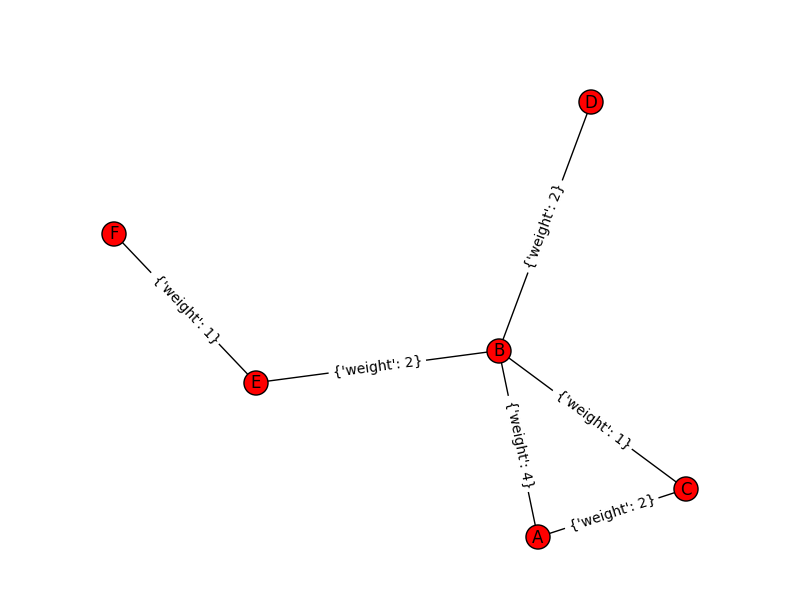# Finding subgroups in a graph using NetworkX and SPSS

This is a task I’ve have to conduct under several guises in the past. Given a set of edges, reduce those edges into unique subgroups based on the transitive closure of those edges. That is, find a group in which all nodes can reach one another (via however many steps are necessary) but are completely separated from all other nodes.

This is steeped in some network language jargon, so I will give a few examples in data analysis where this might be useful:

• Find cliques of offenders (that may resemble a gang) given a set of co-offenders in a police incident database.
• Reduce a large set of items that appear together into smaller subsets. An example may be if you have a multiple response set with a very large number of possible choices. You may identify subgroups of items that occur together.
• Given a set of linked near match names, reduce the database so all of those near match names share the same unique id.
• For my dissertation I aggregate crime incidents to street midpoints and intersections. This creates some overlap or near overlap points (e.g. at T intersections). You might want to further aggregate points that are within a prespecified distance, but there may be multiple nodes all within a short distance. These create a string of networked locations that are probably not appropriate to simply aggregate – especially when they include a large number of locations.

One (typical) way to find the transitive closure is to represent your edges in a binary adjacency matrix and then take subsequent higher powers of that matrix until the diffusion ceases. This is difficult to impossible though with node lists of any substantial size. In this post what I will do is use the NetworkX python library, which contains a handy function named `components.connected` that solves this problem for me.

So first for illustration lets make a small edge list in SPSS.

``````DATA LIST FREE / A B.
BEGIN DATA
1 2
2 3
3 4
5 6
7 8
4 9
7 9
8 10
END DATA.
DATASET NAME Test.
FORMATS A B (F5.0).
EXECUTE.``````

Now using the functions described in this StackOverflow post, we will be able to turn a set of nested lists into a NetworkX object in python.

``````BEGIN PROGRAM.
import networkx
from networkx.algorithms.components.connected import connected_components

def to_graph(l):
G = networkx.Graph()
for part in l:
# each sublist is a bunch of nodes
# it also imlies a number of edges:
return G

def to_edges(l):
"""
treat `l` as a Graph and returns it's edges
to_edges(['a','b','c','d']) -> [(a,b), (b,c),(c,d)]
"""
it = iter(l)
last = next(it)

for current in it:
yield last, current
last = current
END PROGRAM.``````

Now this python code 1) imports our edge list from the SPSS dataset and turn it into a networkx graph, 2) reduces the set of edges into connected components, 3) makes a new SPSS dataset where each row is a list of those subgraphs, and 4) makes a macro variable to identify the end variable name (for subsequent transformations).

``````DATASET DECLARE Int.
BEGIN PROGRAM.
#grab SPSS data
import spss, spssdata
alldata = spssdata.Spssdata().fetchall()

#turn SPSS data into graph
G = to_graph(alldata)
results = connected_components(G)
print results
ml = max(map(len,results))

#now make an SPSS dataset
spss.StartDataStep()
datasetObj = spss.Dataset(name='Int')
for i in range(ml):
v = 'V' + str(i+1)
datasetObj.varlist.append(v,0)
for j in results:
datasetObj.cases.append(j)
spss.EndDataStep()

#make a macro value to signify the last variable
macroValue=[]
macroName="!VEnd"
macroValue.append('V' + str(ml))
spss.SetMacroValue(macroName, macroValue)
END PROGRAM.``````

Now we can take that subgroup dataset, named `Int`, reshape it so all of the nodes are in one column and has a second column identifying the subgraph to which it belongs, and then merge this info back to the edge dataset named `Test`.

``````DATASET ACTIVATE Int.
COMPUTE Group = \$casenum.
FORMATS Group (F5.0).
VARSTOCASES
/MAKE A FROM V1 TO !VEnd.
FORMATS A (F5.0).
SORT CASES BY A.

DATASET ACTIVATE Test.
SORT CASES BY A.
MATCH FILES FILE = *
/TABLE = 'Int'
/BY A.
EXECUTE.``````

From here we can make some nice sociogram charts of our subgroups. SPSS’s `layout.network` is not very specific about the type of layout algorithm, but it does a good job here laying out a nice planar graph.

``````GGRAPH
/GRAPHDATASET NAME="edges" DATASET = "Test" VARIABLES=A B Group
/GRAPHDATASET NAME="nodes" DATASET = "Int" VARIABLES=A Group
/GRAPHSPEC SOURCE=INLINE.
BEGIN GPL
SOURCE: e=userSource(id("edges"))
DATA: Ae=col(source(e), name("A"), unit.category())
DATA: Be=col(source(e), name("B"), unit.category())
DATA: Groupe=col(source(e), name("Group"), unit.category())
SOURCE: n=userSource(id("nodes"))
DATA: An=col(source(n), name("A"), unit.category())
DATA: Groupn=col(source(n), name("Group"), unit.category())
GUIDE: axis(dim(1), null())
GUIDE: axis(dim(2), null())
GUIDE: legend(aesthetic(aesthetic.color.interior), null())
ELEMENT: edge(position(layout.network(node(An), from(Ae), to(Be))))
ELEMENT: point(position(layout.network(node(An), from(Ae), to(Be))), color.interior(Groupn), size(size."14"), label(An))
END GPL.``````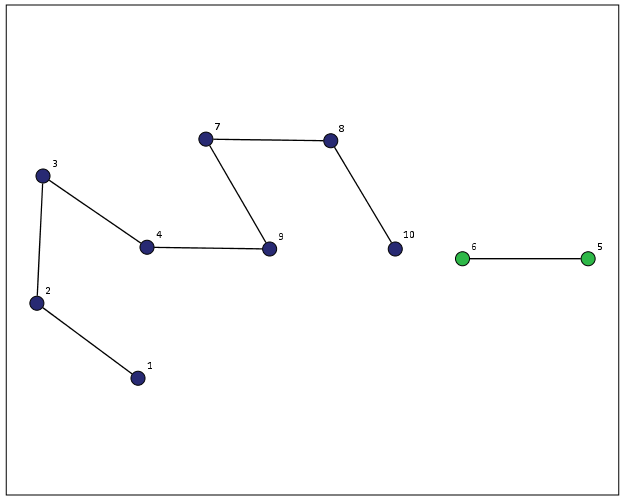At the end of the post I have some more code to illustrate this with a slightly larger random network of 300 potential nodes and 100 random edges. Again SPSS does quite a nice job of laying out the graph, and the colors by group reinforce that our solution is correct.My most recent use application for this had a set of 2,000+ plus edges and this code returned the solution instantaneously. Definitely a speed improvement over taking powers of a binary adjacency matrix in `MATRIX` code.

I wanted to make this network graph using small multiples by group, but I can’t figure out the correct code for the faceting (example commented out at the end of the code snippet). So if anyone has an example of making an SPSS graph with small multiples let me know.

``````*Similar graphs for larger network.
DATASET CLOSE ALL.
INPUT PROGRAM.
COMPUTE #Nodes = 300.
LOOP #i = 1 TO 100.
COMPUTE A = TRUNC(RV.UNIFORM(0,#Nodes+1)).
COMPUTE B = TRUNC(RV.UNIFORM(0,#Nodes+1)).
END CASE.
END LOOP.
END FILE.
END INPUT PROGRAM.
DATASET NAME Test.
FORMATS A B (F5.0).
EXECUTE.

DATASET DECLARE Int.
BEGIN PROGRAM.
#grab SPSS data
import spss, spssdata
alldata = spssdata.Spssdata().fetchall()

#turning SPSS data into NetworkX graph
G = to_graph(alldata)
results = connected_components(G)
ml = max(map(len,results))
print ml

#now make an SPSS dataset
spss.StartDataStep()
datasetObj = spss.Dataset(name='Int')
for i in range(ml):
v = 'V' + str(i+1)
datasetObj.varlist.append(v,0)
for j in results:
datasetObj.cases.append(j)
spss.EndDataStep()

#make a macro value to signify the last variable
macroValue=[]
macroName="!VEnd"
macroValue.append('V' + str(ml))
spss.SetMacroValue(macroName, macroValue)
END PROGRAM.

*Now merging groups back into original set.
DATASET ACTIVATE Int.
COMPUTE Group = \$casenum.
FORMATS Group (F5.0).
VARSTOCASES
/MAKE A FROM V1 TO !VEnd.
FORMATS A (F5.0).
SORT CASES BY A.

DATASET ACTIVATE Test.
SORT CASES BY A.
MATCH FILES FILE = *
/TABLE = 'Int'
/BY A.
EXECUTE.

GGRAPH
/GRAPHDATASET NAME="edges" DATASET = "Test" VARIABLES=A B Group
/GRAPHDATASET NAME="nodes" DATASET = "Int" VARIABLES=A Group
/GRAPHSPEC SOURCE=INLINE.
BEGIN GPL
SOURCE: e=userSource(id("edges"))
DATA: Ae=col(source(e), name("A"), unit.category())
DATA: Be=col(source(e), name("B"), unit.category())
DATA: Groupe=col(source(e), name("Group"), unit.category())
SOURCE: n=userSource(id("nodes"))
DATA: An=col(source(n), name("A"), unit.category())
DATA: Groupn=col(source(n), name("Group"), unit.category())
GUIDE: axis(dim(1), null())
GUIDE: axis(dim(2), null())
GUIDE: legend(aesthetic(aesthetic.color.interior), null())
ELEMENT: edge(position(layout.network(node(An), from(Ae), to(Be))))
ELEMENT: point(position(layout.network(node(An), from(Ae), to(Be))), color.interior(Groupn), size(size."11"))
END GPL.

*This small multiple faceting is not working.
*Error is Groupe & Groupn are not same faceting structure.
* GGRAPH
/GRAPHDATASET NAME="edges" DATASET = "Test" VARIABLES=A B Group
/GRAPHDATASET NAME="nodes" DATASET = "Int" VARIABLES=A Group
/GRAPHSPEC SOURCE=INLINE.
* BEGIN GPL
SOURCE: e=userSource(id("edges"))
DATA: Ae=col(source(e), name("A"), unit.category())
DATA: Be=col(source(e), name("B"), unit.category())
DATA: Groupe=col(source(e), name("Group"), unit.category())
SOURCE: n=userSource(id("nodes"))
DATA: An=col(source(n), name("A"), unit.category())
DATA: Groupn=col(source(n), name("Group"), unit.category())
GUIDE: axis(dim(1), null())
GUIDE: axis(dim(2), null())
GUIDE: legend(aesthetic(aesthetic.color.interior), null())
ELEMENT: edge(position(layout.network(1*1*Groupe, node(An), from(Ae), to(Be))))
ELEMENT: point(position(layout.network(1*1*Groupn, node(An), from(Ae), to(Be))), color.interior(Groupn), size(size."14"), label(An))
END GPL.``````